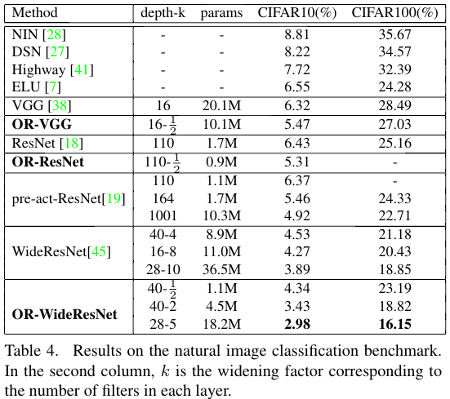## Model

This papers provides a new way to use convolutions. In conventional convolutional neural networks, the filters are only invariant to translation, but the authors propose a new technique to also make them invariant to rotations. This method is called Oriented Response Network (ORN). The ORN is built on top of Active Rotating Filters (ARF).

### ARF

An ARF $$\mathcal{F}$$ is a filter of size $$W \times W \times N$$ where $$N$$ is the number of rotations during the convolution. These produce a feature map of $$N-1$$ orientations. The rotated variant of $$\mathcal{F}$$ called $$\mathcal{F}_\theta$$ are constructed in two steps: coordinate rotation, and orientation spin.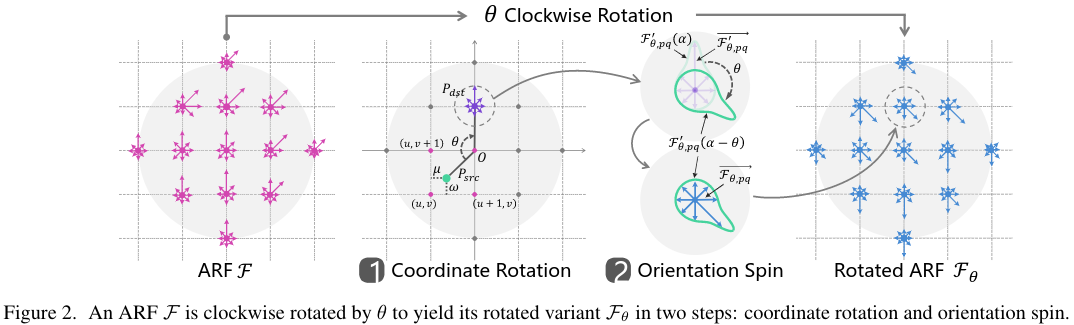To be efficient, the coordinate rotation and orientation spin are calculated by the circular shift operator in the fourier domain.

### Oriented Response Convolution (ORConv)

The ORConv can be seen as a composition of the ARF $$\mathcal{F}$$ and an N-channel input feature map $$\mathcal{M}$$. This combination is denoted as $$\hat\mathcal{M} = \mathbf{ORConv}(\mathcal{F}, \mathcal{M})$$, where $$\hat\mathcal{M}$$ are the output feature maps with $$\mathcal{N}$$ orientations.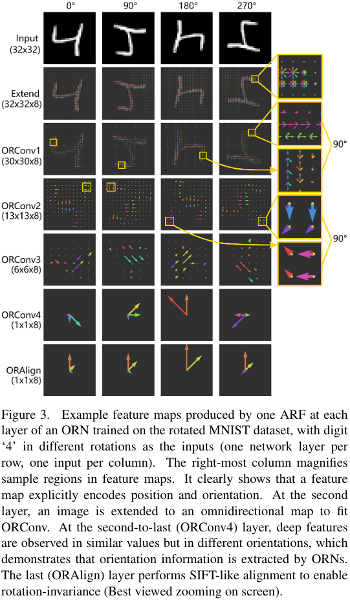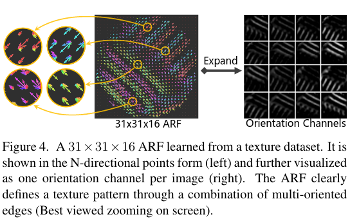### Rotation invariant Feature encoding

By default, the feature maps of ORN are not rotation invariant. When the task needs to be within-class rotation invariant they use two strategies, ORAlign and ORPooling.

Notation: $$\hat\mathcal{M}\{i\}^{(d)}$$ where $$i$$ is the i-th feature map of the ORConv layer, and $$d$$ is the orientation.

#### ORAlign

The ORAlign simply computes the dominant orientation $$\mathcal{D} = \text{argmax}_d \hat\mathcal{M}\{i\}^{(d)}$$ and rotate the feature by $$-\mathcal{D}\frac{2\pi}{N}$$.

#### ORPooling

This pooling consists of simply extracting the maximum orientation of a given feature map $$\text{max}(\hat\mathcal{M}\{i\}^{(d)})$$

## Results

They built an MNIST dataset with rotation from $$[-\frac{\pi}{2}, \frac{\pi}{2}]$$. They report results and show the feature encoding using tSNE for numbers that are similar, like $$6$$ and $$9$$.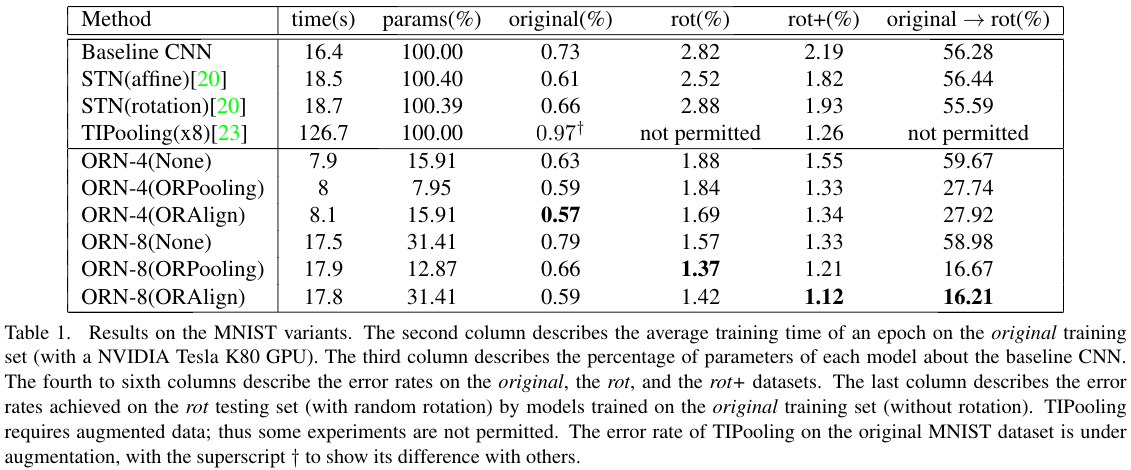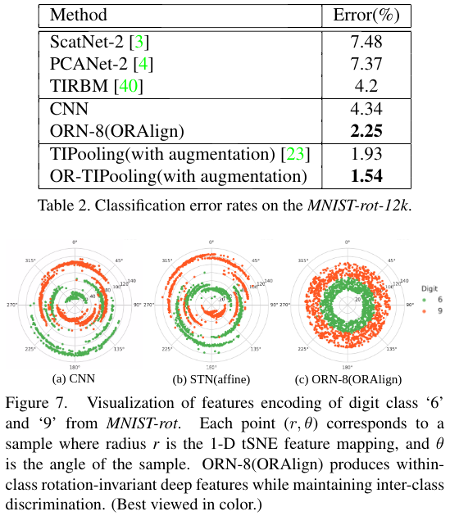They also report results on CIFAR10 dataset and produce better results with less parameters.